# Line plot for two-way designs using ggplot2

Want to use R to plot the means and compare differences between groups, but don’t know where to start? This post is for you.

``````library(dplyr)
library(ggplot2)

pd <- position_dodge(width = 0.2)
mtcars %>%
mutate(cyl = factor(cyl), am = factor(am, labels = c("automatic", "manual"))) %>%
group_by(cyl, am) %>%
summarise(hp_mean = mean(hp),
hp_ci   = 1.96 * sd(hp)/sqrt(n())) %>%
ggplot(aes(x = cyl, y = hp_mean, group = am)) +
geom_line(aes(linetype = am), position = pd) +
geom_errorbar(aes(ymin = hp_mean - hp_ci, ymax = hp_mean + hp_ci),
width = .1, position = pd, linetype = 1) +
geom_point(size = 4, position = pd) +
geom_point(size = 3, position = pd, color = "white") +
guides(linetype = guide_legend("Transmission")) +
labs(title = paste("Mean horsepower depending on",
"number of cylinders and transmission type.",
"Error bars represent 95% Confidence Intervals",
sep = "\n"),
x = "Number of cylinders",
y = "Gross horsepower") +
theme(
panel.background = element_rect(fill = "white"),
legend.key  = element_rect(fill = "white"),
axis.line.x = element_line(colour = "black", size = 1),
axis.line.y = element_line(colour = "black", size = 1)
)
``````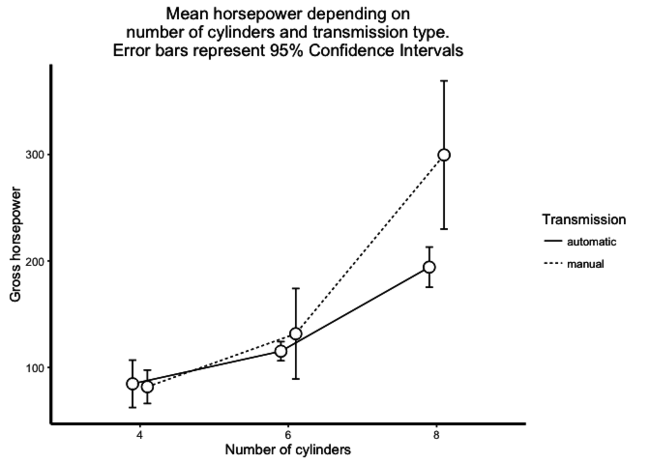Let’s break this down.

## Summarising the data #

The first challenge is the data. When attempting to make a plot like this in R, I’ve noticed that many people (myself included) start by searching for how to make line plots, etc. in R. This is natural. However, for those who are relatively new to R and are more comfortable with the likes of SPSS, being able to produce the plot isn’t necessarily the place to start. Rather, the first thing you should think about is transforming your data into the points that are going to be plotted.

Imagine the plot you’re about to produce. In our example, each point represents the mean horsepower of some group (based on the number of cylinders and transmission), and error bars represent the 95% confidence intervals. We’re not plotting every point in our data set; we’re plotting very specific summary statistics. So, although programmes like SPSS do this summary behind the scenes for us (and there are ways to make this happen automatically in R), I find that it’s best to explicitly calculate these values as a sanity check and to better understand our data. Let’s get to it.

We’ll be working with the `mtcars` dataset which comes with R and contains various information about 32 cars from 1974. Run `?mtcars` from your console to learn more. Here, we’re interested in plotting the mean gross horsepower (`hp`) as a function of two categorical variables: the number of cylinders (`cyl`) and the transmission type (`am`). As a quick aside, note that this example will, therefore, be directly applicable to plotting the mean of a continuous variable grouped by two categorical variables (e.g., from a two-way experimental design).

Let’s focus on the relevant columns and convert the grouping variables to factors. To do this, we’ll use `select()` and `mutate()` from the `dplyr` package. We won’t bother giving `cyl` labels (as they’re already the numbers we want), but we’ll label `am` so we don’t need to worry about converting 0s and 1s in our head.

``````library(dplyr)
d <- mtcars %>%
select(cyl, am, hp) %>%  # select relevant variables
mutate(cyl = factor(cyl),  # Convert grouping variables to factors
am  = factor(am, labels = c("automatic", "manual")))

#>   cyl        am  hp
#> 1   6    manual 110
#> 2   6    manual 110
#> 3   4    manual  93
#> 4   6 automatic 110
#> 5   8 automatic 175
#> 6   6 automatic 105
``````

We need the mean horsepower for each cylinder-transmission combination. This is best achieved with a couple more functions from `dplyr`: group the data by the factors with `group_by()`, and `summarise()` to compute the means:

``````d %>%
group_by(cyl, am) %>%
summarise(hp_mean = mean(hp))
#> Source: local data frame [6 x 3]
#> Groups: cyl [?]
#>
#>      cyl        am   hp_mean
#>   <fctr>    <fctr>     <dbl>
#> 1      4 automatic  84.66667
#> 2      4    manual  81.87500
#> 3      6 automatic 115.25000
#> 4      6    manual 131.66667
#> 5      8 automatic 194.16667
#> 6      8    manual 299.50000
``````

We now have a mean column which represents where the points will go on in the plot. Let’s also compute the distance from the mean to the 95% confidence interval (`hp_ci`) in anticipation of the error bars and save the resulting data frame:

``````sum_d <- d %>%
group_by(cyl, am) %>%
summarise(hp_mean = mean(hp),
hp_ci   = 1.96 * sd(hp)/sqrt(n()))
sum_d
#> Source: local data frame [6 x 4]
#> Groups: cyl [?]
#>
#>      cyl        am   hp_mean     hp_ci
#>   <fctr>    <fctr>     <dbl>     <dbl>
#> 1      4 automatic  84.66667 22.242138
#> 2      4    manual  81.87500 15.699402
#> 3      6 automatic 115.25000  8.995204
#> 4      6    manual 131.66667 42.466667
#> 5      8 automatic 194.16667 18.875105
#> 6      8    manual 299.50000 69.580000
``````

For those a little rusty with statistics, `sd(hp)/sqrt(n())` gives us the standard error of the mean, and multiplying this by 1.96 gives us the distance from the mean to the 95% interval boundary (which we then add or subtract from the mean).

Great, we’ve now got a data frame of the information we need to produce the plot.

## Creating the plot #

To create the plot, we’ll use the `ggplot2` package. When you create a plot with `ggplot2`, you build up layers of graphics. It’s important to keep this idea of layering in mind as we gradually build the plot.

### Setup #

To start, we’ll set up a blank plot canvas with relevant x and y-axes using `ggplot()`:

``````sum_d %>%
ggplot(aes(x = cyl, y = hp_mean))
``````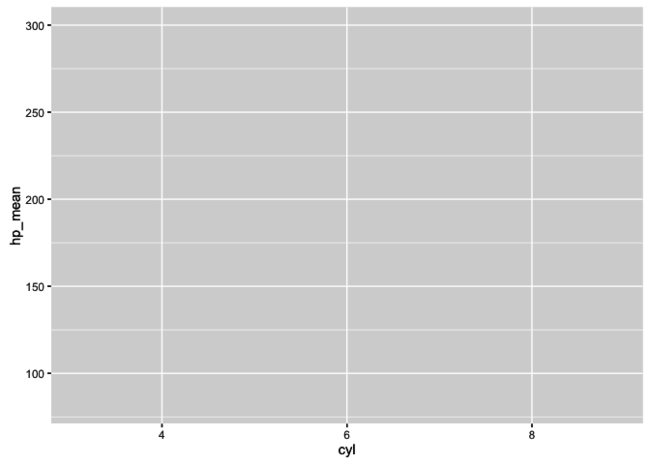Next, we want to represent our data in our plot. `ggplot2` uses various geoms to do this, which are layered into the plot using `+`. Let’s start with the points themselves by using `geom_point()`:

``````sum_d %>%
ggplot(aes(x = cyl, y = hp_mean)) +
geom_point()
``````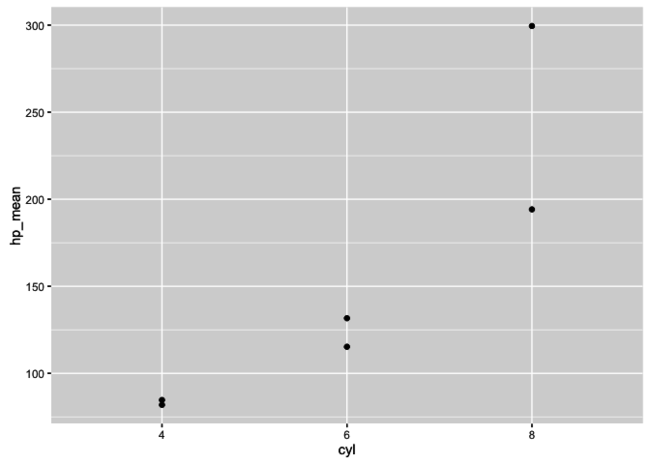Next we’ll add the lines with `geom_line()`:

``````sum_d %>%
ggplot(aes(x = cyl, y = hp_mean)) +
geom_point() +
geom_line()
``````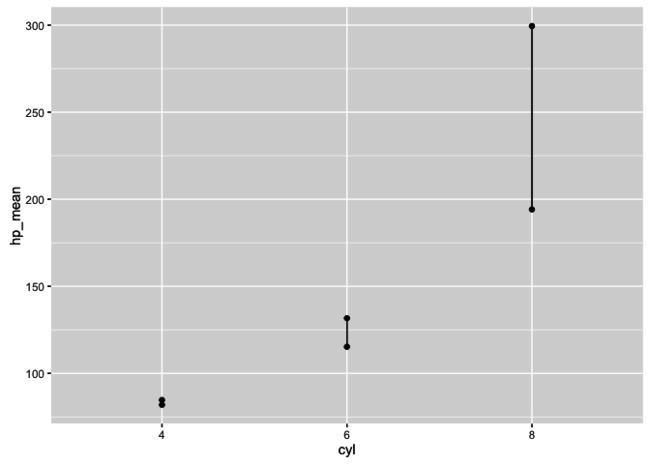Hmm, that doesn’t seem right! What’s going on here? The problem is that we haven’t specified that the lines should be grouped by transmission, so it’s just using the already provided number of cylinders. To handle this, we assign the `group` and `linetype` aesthetics to our second categorical variable, `am`. Note that `group` is handled in `ggplot`, but `linetype` is in `geom_line()`. These can be moved around, but having `group` in `ggplot` is important for the position adjustment discussed later.

``````sum_d %>%
ggplot(aes(x = cyl, y = hp_mean, group = am)) +
geom_point() +
geom_line(aes(linetype = am))
``````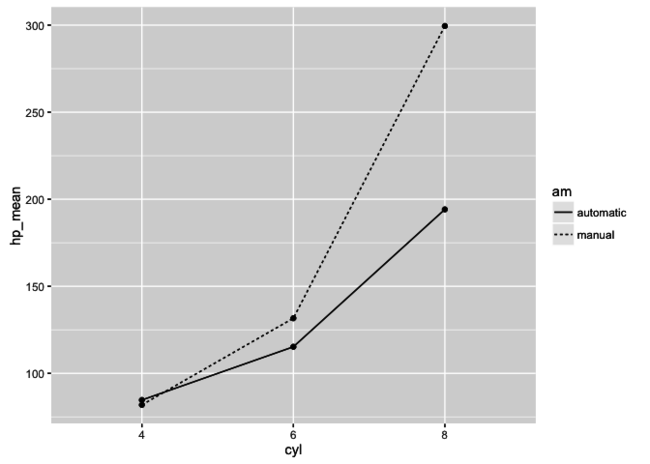The last visual element to add is the error bars using `geom_errorbar()`, which requires us to specify `ymin` and `ymax` for each error bar. I like to do the calculation within this function as follows:

``````sum_d %>%
ggplot(aes(x = cyl, y = hp_mean, group = am)) +
geom_point() +
geom_line(aes(linetype = am)) +
geom_errorbar(aes(ymin = hp_mean - hp_ci, ymax = hp_mean + hp_ci))
``````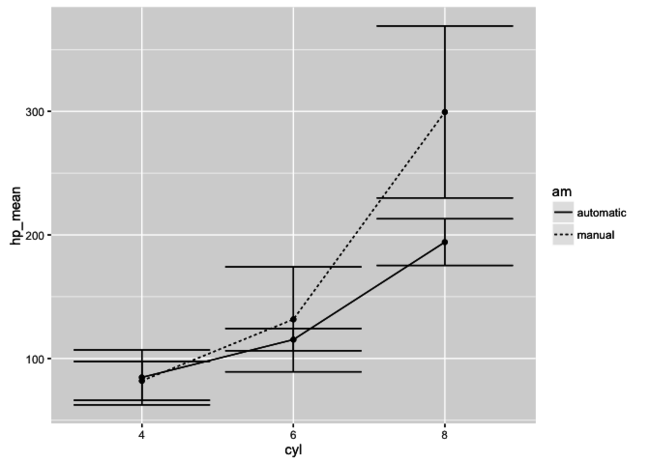OK, not looking perfect yet, but let’s quickly discuss how the error bars are working. By declaring `ymin = hp_mean - hp_ci`, we’re saying that the minimum level of the error bar for each point will be `hp_mean` (which is the position of the point itself) minus the distance to the confidence interval boundary (one standard error multiplied by 1.96). For `ymax`, we add rather than subtract, thus giving us the upper bound for the 95% confidence interval. A quick note, you can change these error bar values to whatever suits you (e.g., could drop `* 1.96` from the original calculation for a single standard error).

## Make it pretty #

We’ve got all the visual elements we need. Now, it’s all about making the plot look nice.

### Error bars #

Let’s start by making those error bars a little less intense by reducing their `width`:

``````sum_d %>%
ggplot(aes(x = cyl, y = hp_mean, group = am)) +
geom_point() +
geom_line(aes(linetype = am)) +
geom_errorbar(aes(ymin = hp_mean - hp_ci, ymax = hp_mean + hp_ci),
width = .1)  # Reduce the width of the error bars
``````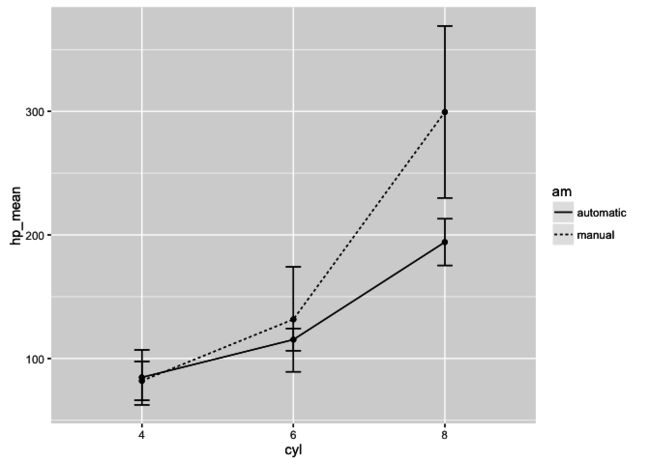### Points #

Next, let’s get the points to be big white circles with black outlines. We’ll start by adjusting the `size` and `color` of the points:

``````sum_d %>%
ggplot(aes(x = cyl, y = hp_mean, group = am)) +
geom_point(size = 3, color = "white") +  # Increase point size and change to white.
geom_line(aes(linetype = am)) +
geom_errorbar(aes(ymin = hp_mean - hp_ci, ymax = hp_mean + hp_ci),
width = .1)
``````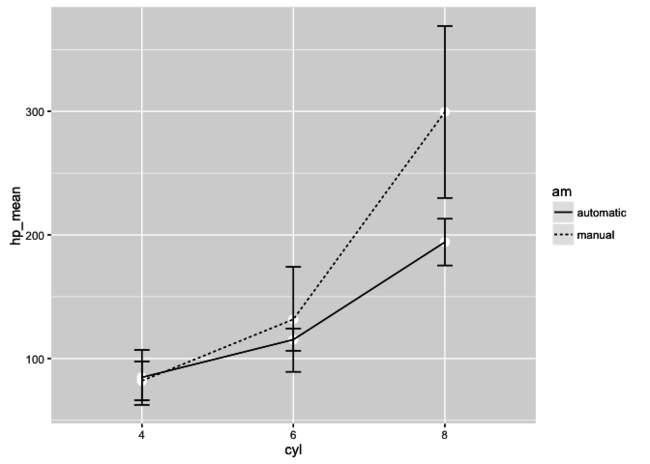What’s gone wrong here? Well, a couple of things. Firstly, the points are being obscured by the lines and error bars. Secondly, the points don’t have a black outline. Handling the first problem is easier. Recall that we’re building the plot up with layers. So `ggplot2` places each layer on top of previous ones. Thus, the order in which we create the geoms will determine which layers fall on top of the others. We created the `geom_point()` layer before `geom_line()` and `geom_errorbar()`, so the points appear behind them. Therefore, the fix is easy – add the points AFTER the other layers as such:

``````sum_d %>%
ggplot(aes(x = cyl, y = hp_mean, group = am)) +
geom_line(aes(linetype = am)) +
geom_errorbar(aes(ymin = hp_mean - hp_ci, ymax = hp_mean + hp_ci),
width = .1) +
geom_point(size = 3, color = "white")
``````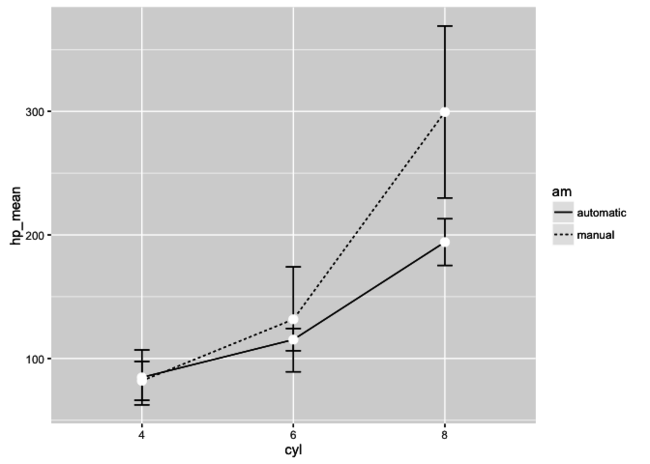Now, what about those black outlines? Thanks to the layering, we can put black points, which are slightly larger in `size` than the white points, in the plot before, and thus behind, the white points. Doing this creates the effect that the points have an outline. Admittedly, this is a little bit of trickery for which a better approach might exist, but this is how I do it:

``````sum_d %>%
ggplot(aes(x = cyl, y = hp_mean, group = am)) +
geom_line(aes(linetype = am)) +
geom_errorbar(aes(ymin = hp_mean - hp_ci, ymax = hp_mean + hp_ci),
width = .1) +
geom_point(size = 4) +  # Add black points first; larger size than white points
geom_point(size = 3, color = "white")
``````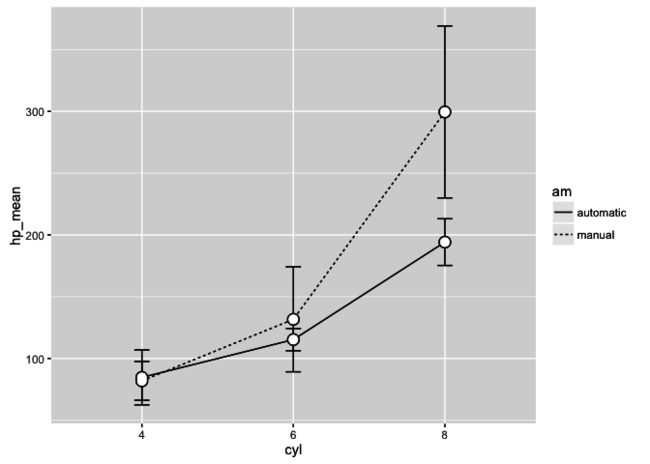If you were to comment out the last line of the above code, you’ll see that it’s just large black points that are being plotted, and over which we’re putting smaller white ones.

### Position #

Next, we’ll adjust the `position` of the points so that they, and their error bars, don’t overlap. First, remember that there are multiple geom layers: the lines, the error bars, and two point layers. Each of these has to be adjusted separately. We can do this inside our pipe, but to save the effort when tweaking the value, let’s set a single adjustment variable as follows:

``````pd <- position_dodge(width = 0.2)
``````

Now, we add `position = pd` into all the geom layers:

``````sum_d %>%
ggplot(aes(x = cyl, y = hp_mean, group = am)) +
geom_line(aes(linetype = am), position = pd) +
geom_errorbar(aes(ymin = hp_mean - hp_ci, ymax = hp_mean + hp_ci),
width = .1, position = pd) +
geom_point(size = 4, position = pd) +
geom_point(size = 3, color = "white", position = pd)
``````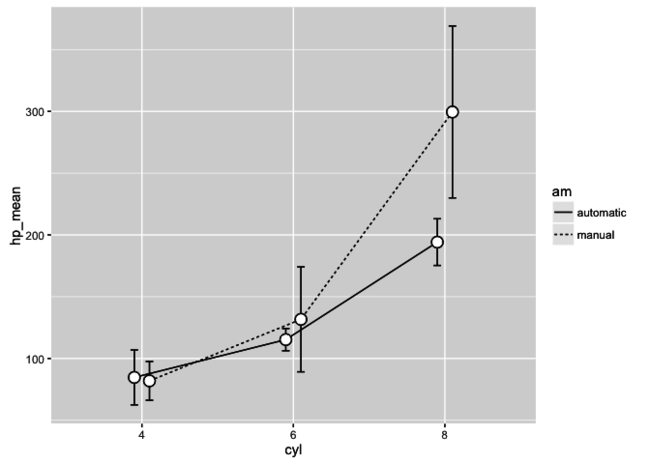### Labels #

Almost there. Now, we need to fix the labels of the axes and the legend and add a title. The axes and title can be handled together using `labs()`. The legend, however, needs to be managed separately using `guides()`. To create a multi-line title, I use `paste()`, and separate each section (`sep =`) of text with `"\n"`, which represents a new line.

``````sum_d %>%
ggplot(aes(x = cyl, y = hp_mean, group = am)) +
geom_line(aes(linetype = am), position = pd) +
geom_errorbar(aes(ymin = hp_mean - hp_ci, ymax = hp_mean + hp_ci),
width = .1, position = pd) +
geom_point(size = 4, position = pd) +
geom_point(size = 3, color = "white", position = pd) +
guides(linetype = guide_legend("Transmission")) +   # Change the legend title
labs(title = paste("Mean horsepower depending on",  # Add a multi-line title
"number of cylinders and transmission type.",
"Error bars represent 95% Confidence Intervals",
sep = "\n"),
x = "Number of cylinders",  # Change x-axis label
y = "Gross horsepower")     # Change y-axis label
``````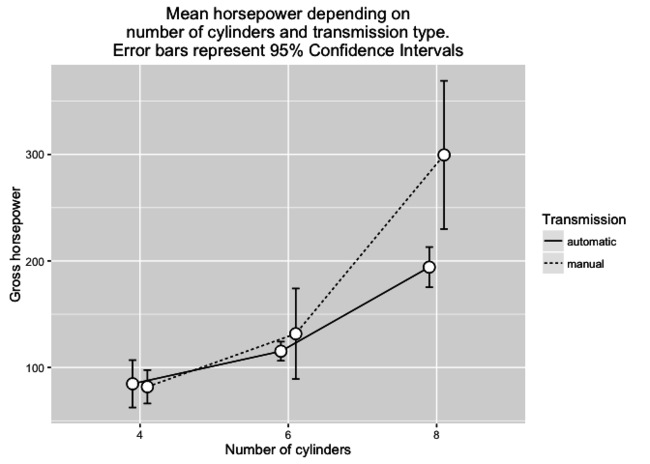### Theme #

The final piece of the puzzle is to add a theme that removes the background grid and adds some solid lines to the x and y-axes:

``````sum_d %>%
ggplot(aes(x = cyl, y = hp_mean, group = am)) +
geom_line(aes(linetype = am), position = pd) +
geom_errorbar(aes(ymin = hp_mean - hp_ci, ymax = hp_mean + hp_ci),
width = .1, position = pd) +
geom_point(size = 4, position = pd) +
geom_point(size = 3, color = "white", position = pd) +
guides(linetype = guide_legend("Transmission")) +
labs(title = paste("Mean horsepower depending on",
"number of cylinders and transmission type",
"Error bars represent 95% Confidence Intervals",
sep = "\n"),
x = "Number of cylinders",
y = "Gross horsepower") +
theme(
panel.background = element_rect(fill = "white"),         # Set plot background to white
legend.key  = element_rect(fill = "white"),              # Set legend item backgrounds to white
axis.line.x = element_line(colour = "black", size = 1),  # Add line to x axis
axis.line.y = element_line(colour = "black", size = 1)   # Add line to y axis
)
``````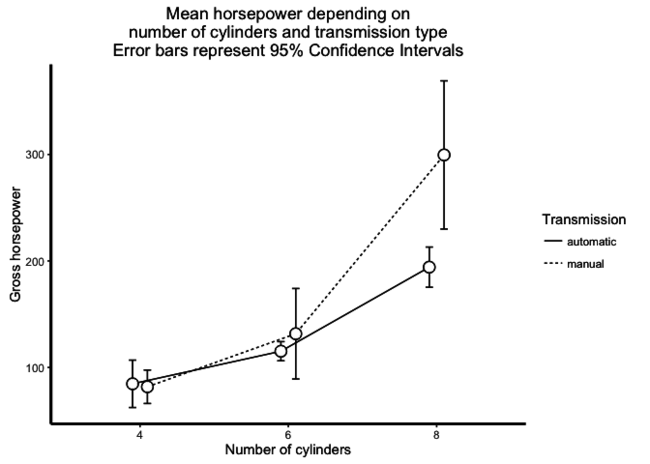The only difference between this and the example at the beginning is that the data preparation (computing mean and confidence interval distance) is handled within a single pipe.

## Sign off #

Thanks for reading and I hope this was useful for you.

For updates of recent blog posts, follow @drsimonj on Twitter, or email me at drsimonjackson@gmail.com to get in touch.

If you’d like the code that produced this blog, check out my GitHub repository, blogR.